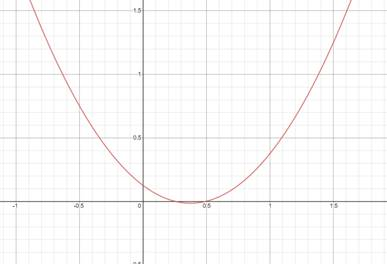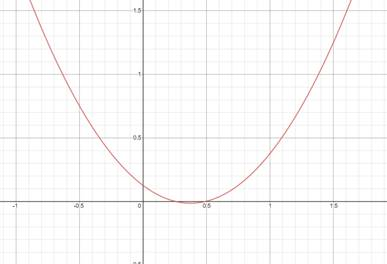# Solve the equation graphically in the given interval.### Precalculus: Mathematics for Calcu...

6th Edition
Stewart + 5 others
Publisher: Cengage Learning
ISBN: 9780840068071### Precalculus: Mathematics for Calcu...

6th Edition
Stewart + 5 others
Publisher: Cengage Learning
ISBN: 9780840068071

#### Solutions

Chapter 1.9, Problem 44E
To determine

## Solve the equation graphically in the given interval.

Expert Solutionx=0.25,0.5

### Explanation of Solution

Given information:

x20.75x+0.125=0

[2,2]

Formula used:

Plot the graph and approximate the solution of x.

Graphically:

Making Graph of x20.75x+0.125=0 and finding approximate value of x.Graph intersect x-axis at (0.25,0),(0.5,0) in the interval of [2,2]

x=0.25,0.5

### Have a homework question?

Subscribe to bartleby learn! Ask subject matter experts 30 homework questions each month. Plus, you’ll have access to millions of step-by-step textbook answers!